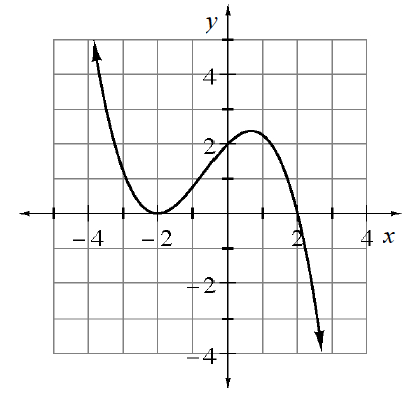### Home > INT3 > Chapter 9 > Lesson 9.1.4 > Problem9-57

9-57.

What is the equation of the graph below?Write the equation by using the $x$-intercepts. Don't forget you have a double root at $x = −2$. Add your constant of proportionality.

$y=k(x+2)(x+2)(x-2)$

Find the constant of proportionality by substituting the $y$-intercept $\left(0, 2\right)$ for $x$ and $y$.

$y =-\frac{1}{4}(x-2)(x+2)^2$